# E-PolyLearning

 1. Which of the following T-H diagram is valid for dehumidification operation ?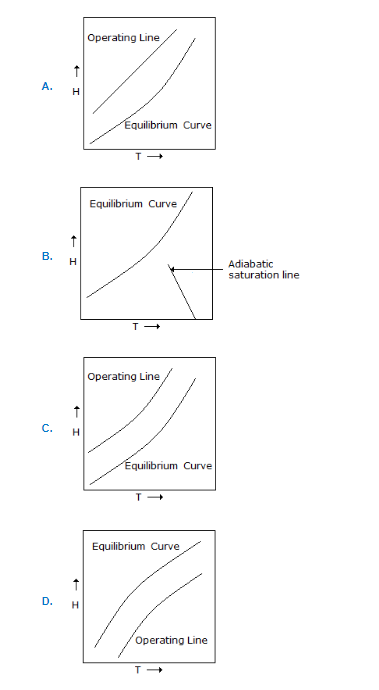a. A b. B c. C d. D

 2. If f = moles of vapour present per mole of feed, then the slope of feed line is (Mcabe-Thiele method)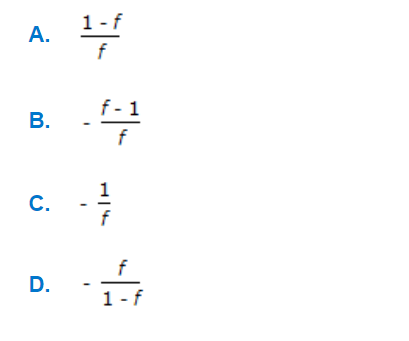a. A b. B c. C d. D
 3. The diffusivity (D) in a binary gas mixture is related to the pressure (P) as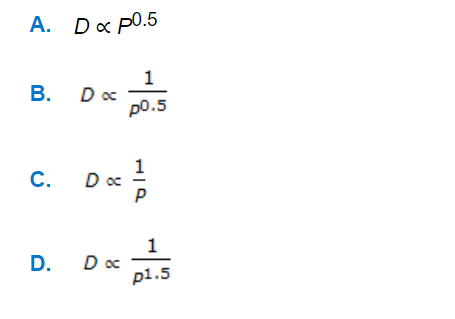a. A b. B c. C d. D
 4. In gas-liquid contact operation, the number of ideal stages N is given by following equation. This is true when the stripping factor 'S' is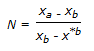a. S > 1 b. S < 1 c. S = 1 d. S = ∞
 5. In a vapor-liquid contacting equipment, the overall gas phase mass transfer co-efficient (M.T.C), KG is related to individual co-efficients (KG and KL) as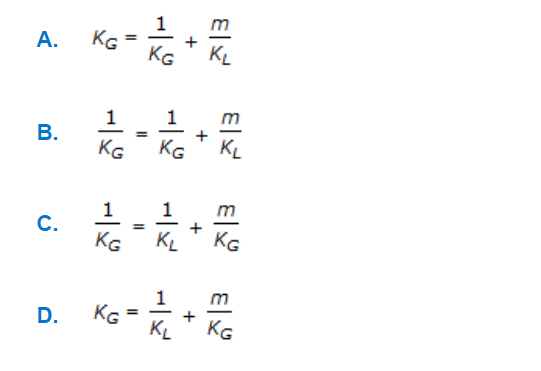a. A b. B c. C d. D